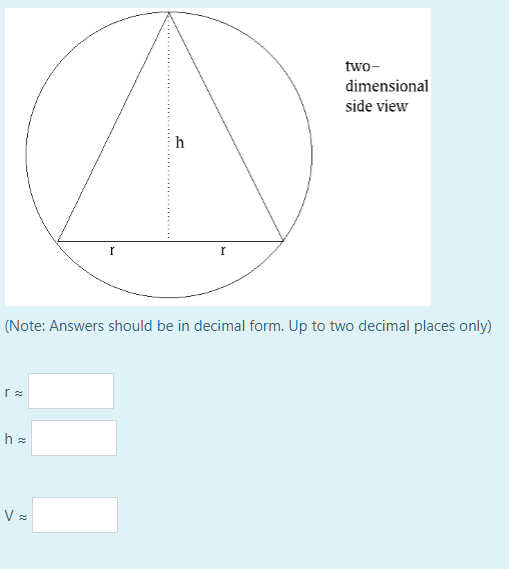find dimensions

 Your name to display (optional): Email me at this address if my answer is selected or commented on: Privacy: Your email address will only be used for sending these notifications. Anti-spam verification: To avoid this verification in future, please log in or register.

Label the endpoints of the base of the isosceles triangular cross-section of the cone B and C and the remaining vertex (apex) A. Mark the centre of the circle as O and perpendicular bisector of A to meet BC at N.

ON=√(4-r²); h=AO+ON=2+√(4-r²).

V=⅓πr²h=⅓πr²(2+√(4-r²))=⅔πr²+⅓πr²√(4-r²)

dV/dr=4πr/3+(⅓πr²)(-2r/√(4-r²))+⅔πr√(4-r²)=0 when V is max or min.

dV/dr=4πr/3-⅔πr³/√(4-r²)+⅔πr√(4-r²)=0.

So using the common denominator 3√(4-r²):

4π⁻r√(4-r²)-2πr³+2πr(4-r²)=0, just considering the numerator.

4π⁻r√(4-r²)-4πr³+8πr=0. Divide through by 4πr:

√(4-r²)-r²+2=0,

√(4-r²)=r²-2. Square both sides:

4-r²=r⁴-4r²+4, -r²=r⁴-4r². Divide through by r²:

-1=r²-4, r²=3, r=√3=1.73 units and h=2+√(4-r²)=2+1=3 units.

V=⅓πr²h=(1/3)π(3)(3)=3π=9.42 cubic units.

by Top Rated User (1.0m points)